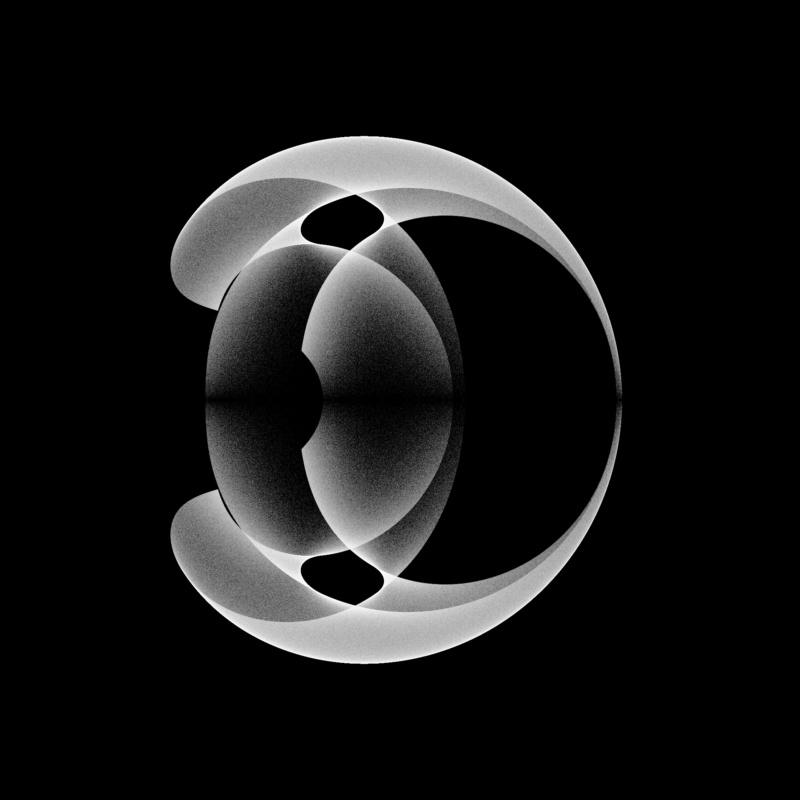# Bohemian MatricesEigenvalues of the matrix $$\begin{bmatrix} 0 & 0 & -1 & -1\\ 1 & -1 & 0 & 0\\ 0 & 1 & A & 0\\ 1 & 0 & -1 & B \end{bmatrix}$$ where $A$ and $B$ are independent continuous uniform random variables on $(-3, 3)$. This image represents a sample of 5 million matrices. Note that this class of matrices is not Bohemian as it is sampled from a continuous distribution. Viewed on [-9/4-2i, 9/4+2i]. Real eigenvalues have been omitted from this plot.